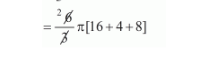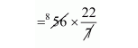# A reservoir is in the shape of a frustum of a right circular cone.`
Question:

A reservoir is in the shape of a frustum of a right circular cone. It is 8 m across at the top and 4 m across at the bottom. If it is 6 m deep, then its capacity is

(a) $176 \mathrm{~m}^{3}$

(b) $196 \mathrm{~m}^{3}$

(c) $200 \mathrm{~m}^{3}$

(d) $110 \mathrm{~m}^{3}$

Solution:

$r_{1}=\frac{8}{2}$

$=4 \mathrm{~m}$

$r_{2}=\frac{4 \mathrm{~m}}{\alpha}$

$=2 \mathrm{~m}$ and

$h=6 \mathrm{~m}$

The volume of reservoir

$=\frac{h}{3} \pi\left\{r_{1}^{2}+r_{2}^{2}+r_{1} r_{2}\right\}$$=56 \pi$

$=56 \pi$$=176 \mathrm{~m}^{2}$

The volume of reservoir = 176 m2

Hence, the correct answer is choice (a).